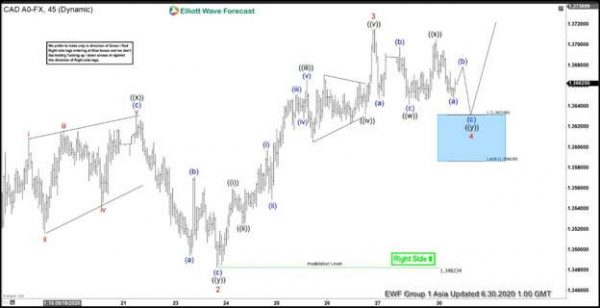USDCAD 45 minutes chart below shows that the pair has ended 3 wave pullback from June 15 high in wave 2. The pullback unfolded as double three Elliott Wave structure and ended at 1.3482 low. Afterwards, the pair resumed higher in wave 3. Up from 1.3482 low, wave ((i)) ended at 1.3559 high. Wave ((ii)) pullback ended at 1.3525 low. The pair then extended higher in wave ((iii)), which ended at 1.3666 high. The internal subwave of wave ((iii)) unfolded as 5 waves impulse Elliott Wave structure in lesser degree. The pullback in wave ((iv)) then unfolded as a triangle and ended at 1.3632 low. From there, the pair pushed higher in wave ((v)) which ended at 1.3715 high. This final move completed wave 3 in higher degree and ended cycle from June 23 low.

The pair is currently doing a pullback in wave 4 to correct the cycle from June 23 low. The correction is unfolding as a double three structure. Down from wave 3 high, the pair ended wave ((w)) at 1.3642 low. Wave ((x)) bounce ended at 1.3705 high. Wave ((y)) is currently in progress. While above 1.3482 low, expect the dips to find support in 7 or 11 swings for more upside. The 100 -161.8% extension of wave ((w))-((x)) where wave ((y)) can potentially end is at 1.3586 – 1.3631 area and shown with a blue box. That area, if reached later, can see a reaction for 3 waves bounce at least.

USD/CAD 45 Minutes Elliott Wave Chart